# Pet The Cat

Aperi'CTF 2019 - Reverse (175 pts).

# Aperi’CTF 2019 - Pet The Cat

### Challenge details

Event Challenge Category Points Solves
Aperi’CTF 2019 Pet The Cat Reverse 175 7

Qu’est-ce que vous faites là ? Allez caresser le chat !

Fichier : - le chat - md5sum: 2cc36435ef2481d785e8d0c4f036f23c

### TL;DR

Crackme compiled with movfuscator to make it hard to reverse. This can still be done statically via demovfuscator or dynamically via a side channel attack. The second method is the one used in this writeup: counting the number of line outputed by ltrace.

### Methodology

First, let’s have a gentle look to its format and behavior…

# Pet The Cat Writeup

Running file on the binary reveals it’s a MachO 64-bit binary.

## Static analysis

Because not everybody has a Mac to run the program on, a static analysis can be performed on this binary.

Let’s open IDA and start dissassembling.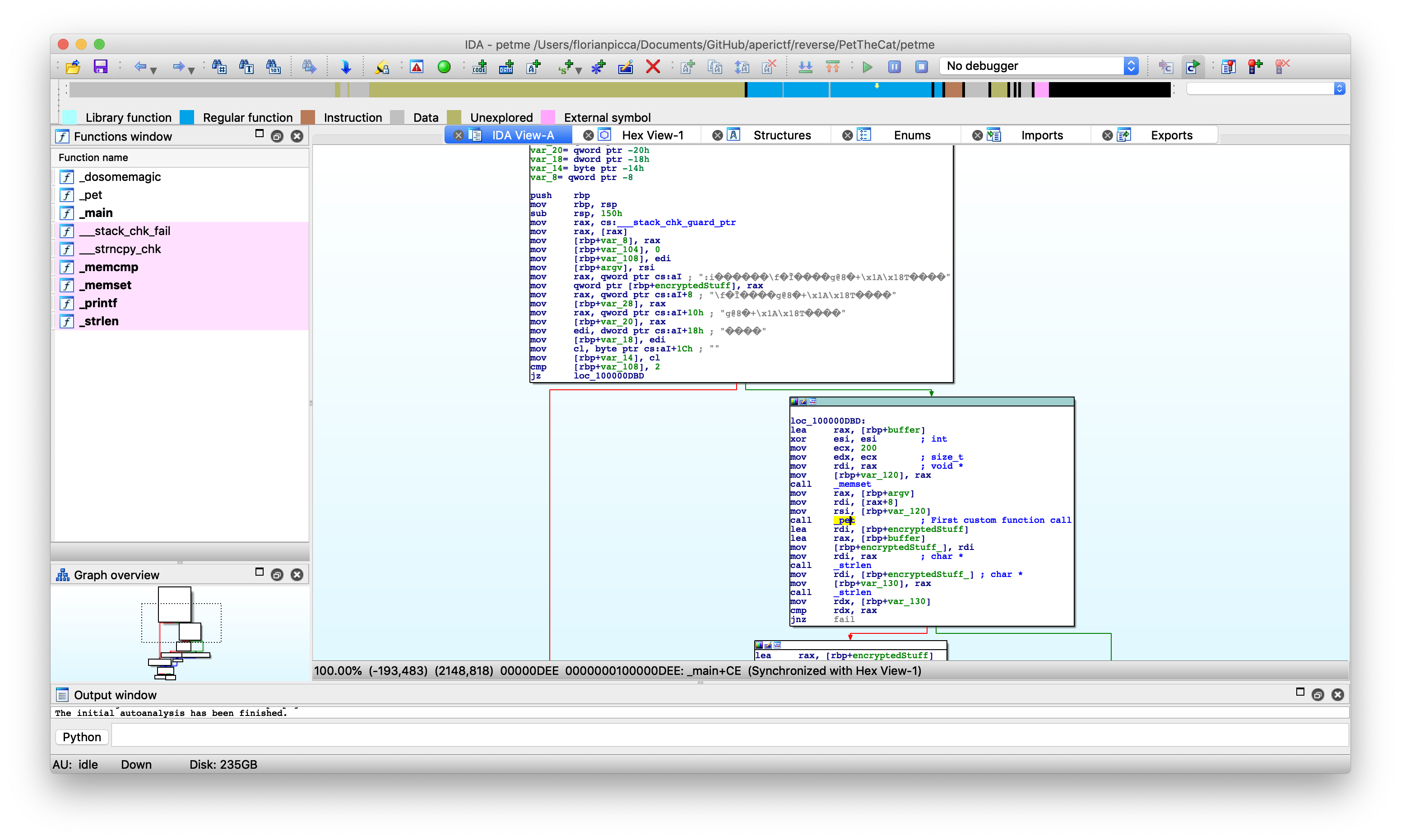This is the main function’s start. Some gribberish can be seen at the start, it must be the flag in encrypted form.

After that a buffer is filled with null bytes and the function pet() is called with 2 arguments :

1. argv
2. The buffer full of nulls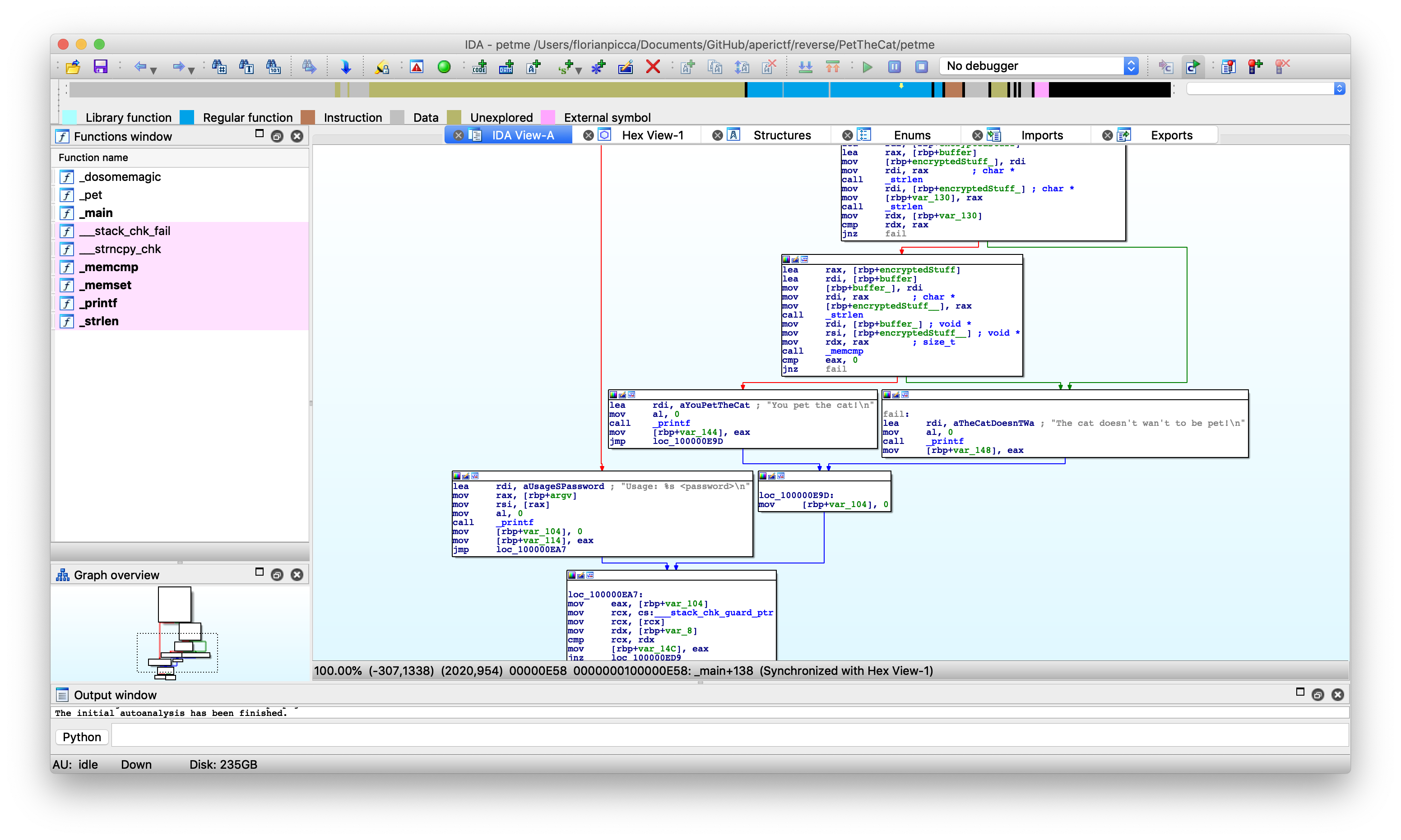Later in the same function, the size of the buffer is compared with the size of the encrypted flag and they must match. If it’s the case and if the buffer is equal to the encrypted flag, the cat is pet. Let’s take a look at what happens inside the previous function.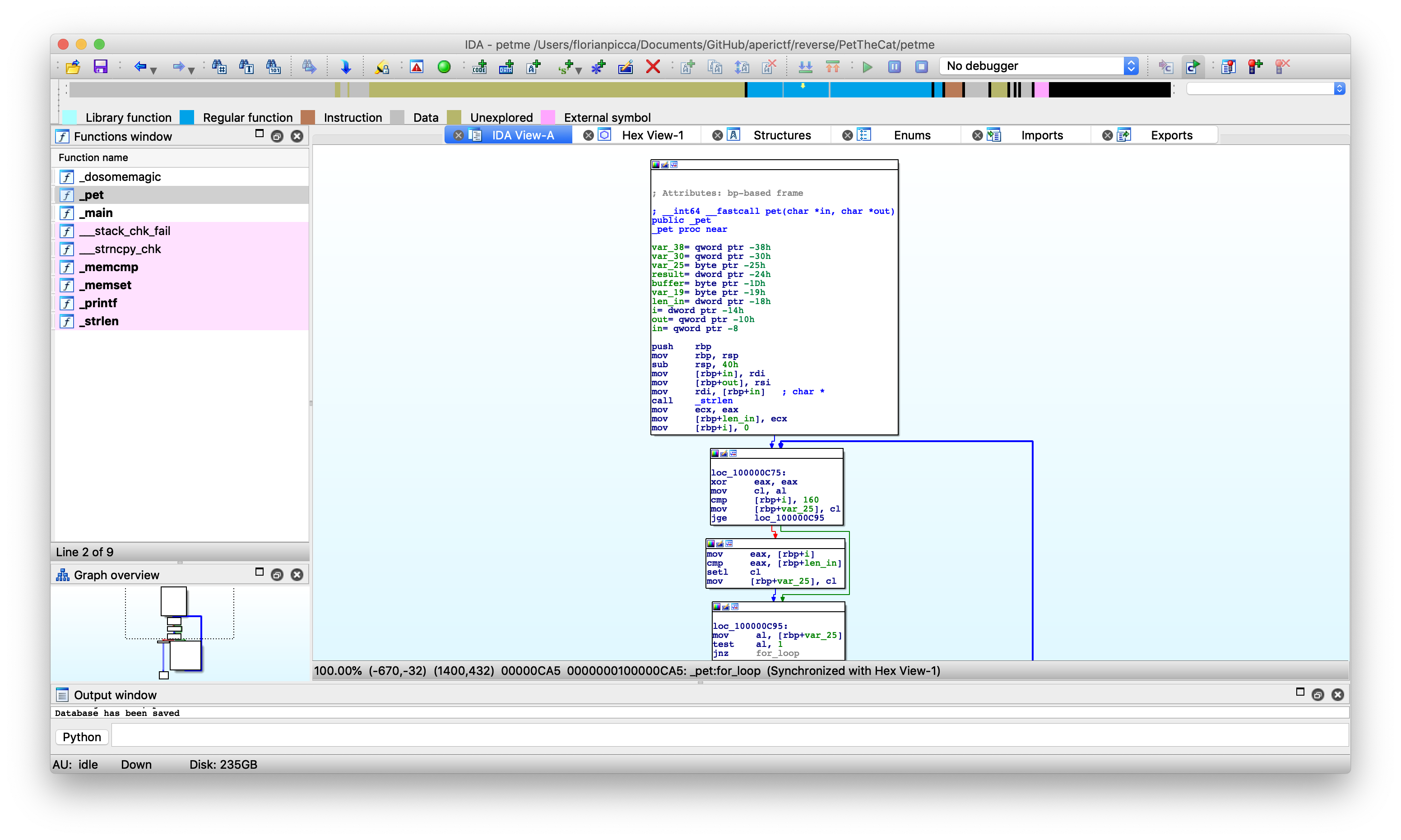Inside the pet() function the size of the first argument is checked before entering what seems to be a for-loop iterating over argv.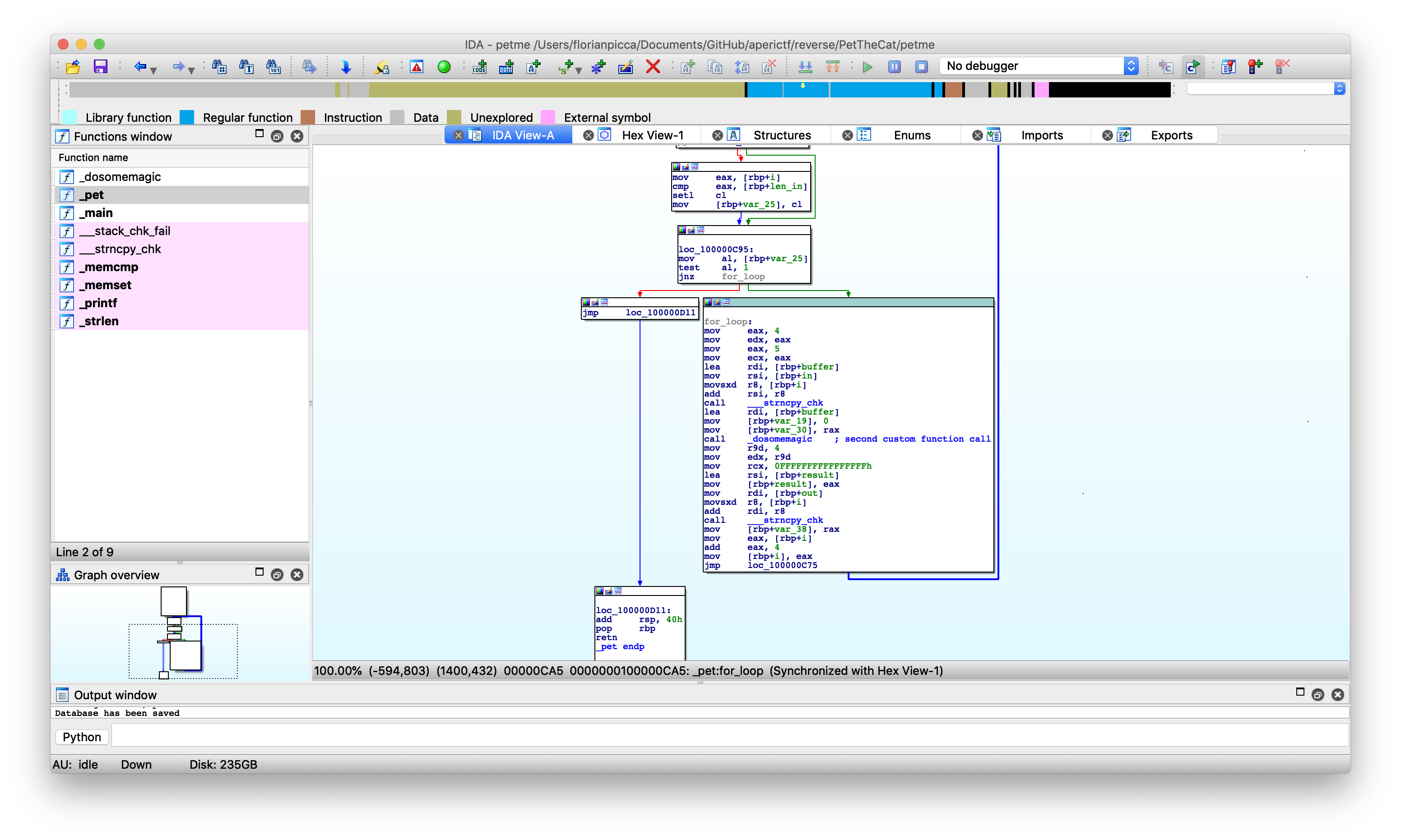The for-loop takes 4 bytes from argv and give them as parameter for the new function dosomemagic(). The result of this function is then copied in the second argument, the previous buffer.

There is only one last function to check.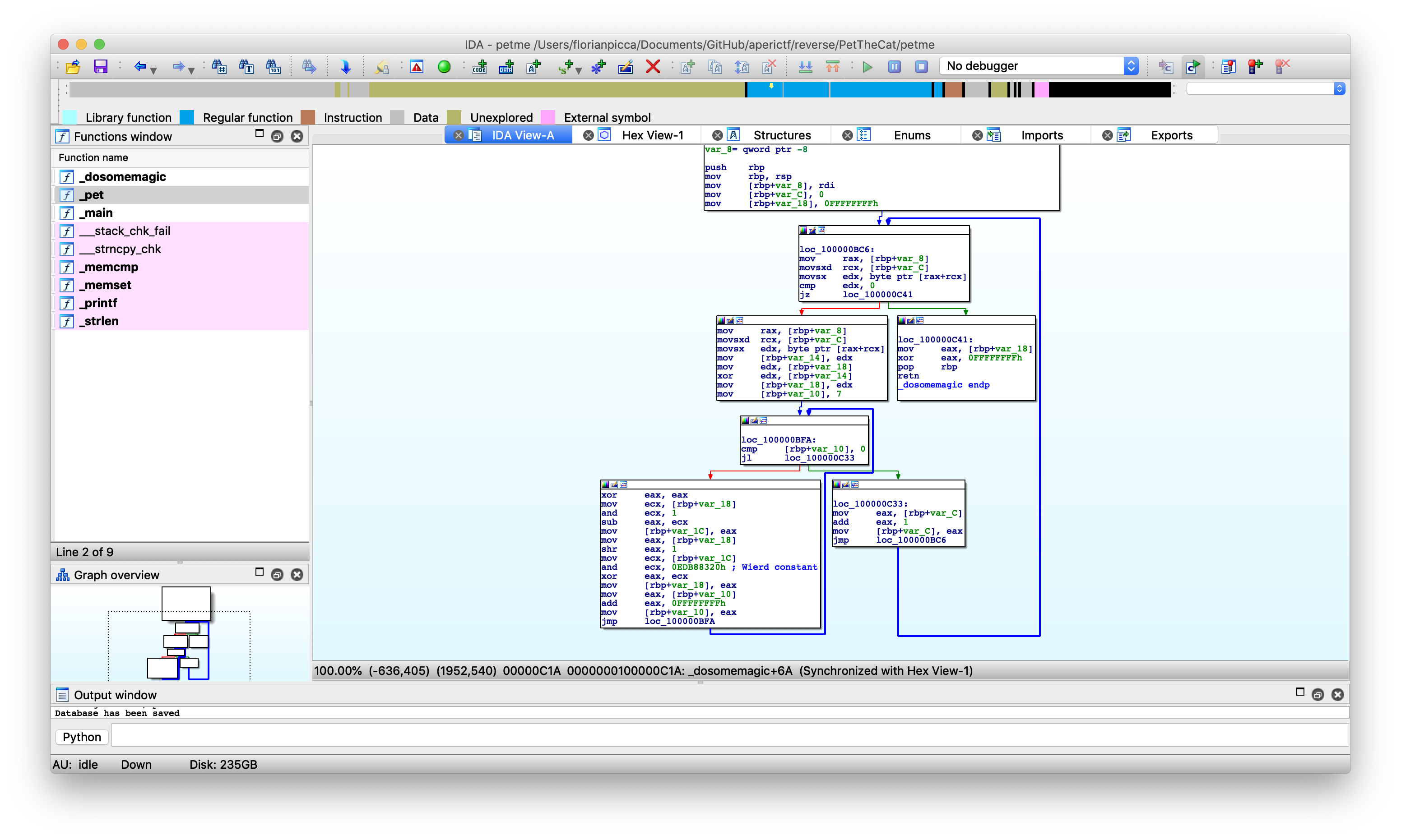This function looks very complicated but there is a strange constant 0xEDB88320. Because this seem to perform some cryptographic transformation, let’s google this value to see if it correspond to any known algorithm.

By doing so, the function can be identified as a CRC32 checksum calculator.

To recap, the password is chunked in blocks of 4 letters and each chunk is replaced by it’s CRC32 checksum.

## Decrypting the flag

Normally CRC32 is not reversible but because the input string is 4 bytes long, it can be done without brute-force.

The encrypted flag extracted from the binary is :

"\x3a\x69\xd3\x07\xe4\xe4\xfe\x88\x0c\xf8\xc8\x88\x81\xc3\xf7\x9d\x67\x40\x38\x9d\x2b\x1a\x18\x54\xf3\x58\xe6\xf0"


Because of the endianness, if we need to convert each chunk to a number, the first two would be :

0x07d3693a	# CRC32("APRK")
0x88fee4e4	# CRC32("{CRC")


After reversing this value and concatenating them we find the flag.

### Full script

Full script available here

#!/usr/bin/env python3
# -*- coding:utf-8 -*-

table = []
table_reverse = []

def init_tables(poly, reverse=True):
global table, table_reverse
table = []
# build CRC32 table
for i in range(256):
for j in range(8):
if i & 1:
i >>= 1
i ^= poly
else:
i >>= 1
table.append(i)
assert len(table) == 256, "table is wrong size"
# build reverse table
if reverse:
table_reverse = []
found_none = set()
found_multiple = set()
for i in range(256):
found = []
for j in range(256):
if table[j] >> 24 == i:
found.append(j)
table_reverse.append(tuple(found))
elif len(found) > 1:
assert len(table_reverse) == 256, "reverse table is wrong size"
if found_multiple:
out('WARNING: Multiple table entries have an MSB in {0}'.format(
rangess(found_multiple)))
if found_none:
out('ERROR: no MSB in the table equals bytes in {0}'.format(
rangess(found_none)))

def findReverse(desired):
solutions = set()
accum = ~0
stack = [(~desired,)]
while stack:
node = stack.pop()
for j in table_reverse[(node >> 24) & 0xFF]:
if len(node) == 4:
a = accum
data = []
node = node[1:] + (j,)
for i in range(3, -1, -1):
data.append((a ^ node[i]) & 0xFF)
a >>= 8
a ^= table[node[i]]
else:
stack.append(((node ^ table[j]) << 8,) + node[1:] + (j,))
return solutions

def solve(wanted):
a = findReverse(wanted)
t = []
for e in a:
s = ""
for l in e:
s += chr(l)
t.append(s)
return t

init_tables(0xEDB88320)
print solve(0x7d3693a) + solve(0x88fee4e4) + solve(0x88c8f80c) + solve(0x9df7c381) + solve(0x9d384067) + solve(0x54181a2b) + solve(0xF0E658F3)


### Flag

APRK{CRC32_1sr3v3rs1bl3;)}

ENOENT